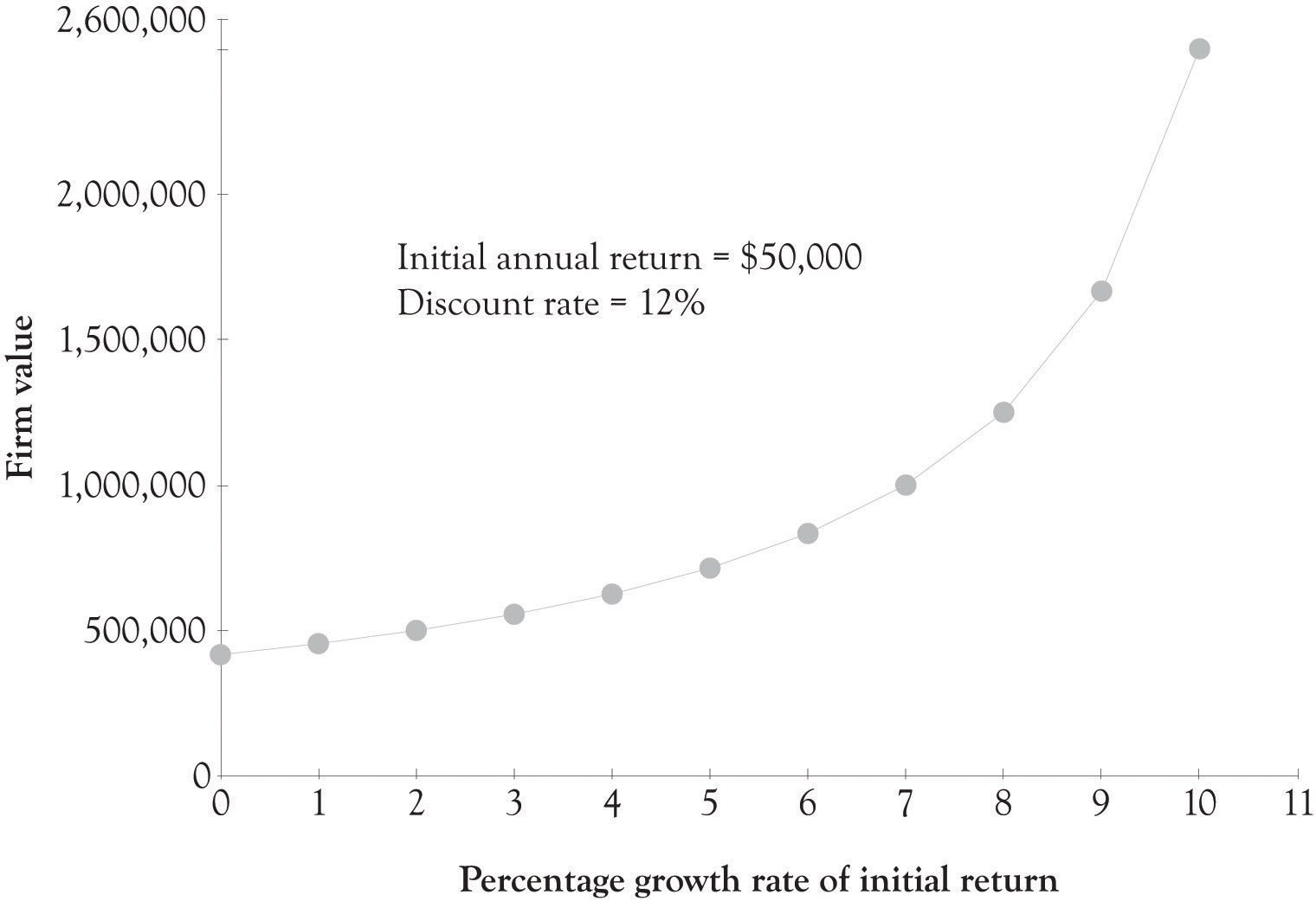This is “The Importance of Growth Rate on Firm Value”, section 11.7 from the book Creating Services and Products (v. 1.0). For details on it (including licensing), click here.

Has this book helped you? Consider passing it on:
Creative Commons supports free culture from music to education. Their licenses helped make this book available to you.
DonorsChoose.org helps people like you help teachers fund their classroom projects, from art supplies to books to calculators.

## 11.7 The Importance of Growth Rate on Firm Value

The growth rate of revenues can have a dramatic impact on the value of the business. As noted earlier, one way to determine the value of a business is to use the formula for the present value of perpetual annuity. The following formula was used.

Present Value of Ordinary Perpetuity = Constant annual return/Discount rate

This formula can be slightly modified to include a growth in annual returns.

Figure 11.1 The Influence of Growth Rate on Firm ValueFirm Value = Initial Annual Return/(Discount Rate − Growth Rate of Initial Return)

If the initial annual return is \$50,000 and the cost of capital is 8% and revenues are not expected to grow then the value of the firm would be \$625,000. If the initial annual return is expected to grow at 2%, then the value of the firm would increase to \$833,333.

Figure 11.1 "The Influence of Growth Rate on Firm Value" presents a graph where the growth rate ranges from 0–10% with a discount rate of 12% and an initial annual return of \$50,000. The present value of a firm with 0% growth rate is approximately \$417,000. The present value of the firm with a 10% growth rate is \$2,500,000. As the growth rate approaches the discount rate the present value of the firm increases exponentially. This in part illustrates why companies with very modest returns are sometimes valued very highly by potential investors. A firm with strong growth potential, even with small initial returns, is an attractive target for investors.